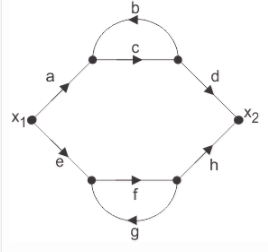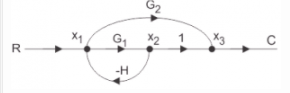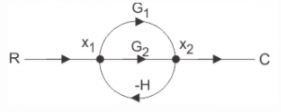Courses

# Test: Signal Flow Graphs

## 15 Questions MCQ Test Mock Tests Electronics & Communication Engineering GATE 2020 | Test: Signal Flow Graphs

Description
This mock test of Test: Signal Flow Graphs for GATE helps you for every GATE entrance exam. This contains 15 Multiple Choice Questions for GATE Test: Signal Flow Graphs (mcq) to study with solutions a complete question bank. The solved questions answers in this Test: Signal Flow Graphs quiz give you a good mix of easy questions and tough questions. GATE students definitely take this Test: Signal Flow Graphs exercise for a better result in the exam. You can find other Test: Signal Flow Graphs extra questions, long questions & short questions for GATE on EduRev as well by searching above.
QUESTION: 1

### A signal flow graph is the graphical representation of the relationships between the variables of set linear algebraic equations.

Solution:

Explanation: By definition signal flow graphs are the graphical representation of the relationships between the variables of set linear algebraic equations.

QUESTION: 2

### A node having only outgoing branches.

Solution:

Explanation: Nodes are the point by which the branches are outgoing or ingoing and this can be input or output node and input node is the node having only outgoing branches.

QUESTION: 3

### Use mason’s gain formula to find the transfer function of the given signal flow graph:

Solution:

Explanation: Using mason’s gain formula transfer function from signal flow graph can be calculated which relates the forward path gain to the various paths and loops.

QUESTION: 4

Use mason’s gain formula to find the transfer function of the following signal flow graph:Solution:

Explanation: Using mason’s gain formula transfer function from signal flow graph can be calculated which relates the forward path gain to the various paths and loops.

QUESTION: 5

Loop which do not possess any common node are said to be ___________ loops.

Solution:

Explanation: Loop is the part of the network in which the branch starts from the node and comes back to the same node and non touching loop must not have any node in common.

QUESTION: 6

Signal flow graphs:

Solution:

Explanation: Signal flow graphs are used to find the transfer function of control system by converting the block diagrams into signal flow graphs or directly but cannot be used for nonlinear systems.

QUESTION: 7

Signal flow graphs are reliable to find transfer function than block diagram reduction technique.

Solution:

Explanation: As one set technique and formula is used here but in block diagram technique various methods are involved which increases complexity.

QUESTION: 8

The relationship between an input and output variable of a signal flow graph is given by the net gain between the input and output node is known as the overall______________

Solution:

Explanation: The relationship between input and output variable of a signal flow graph is the overall gain of the system.

QUESTION: 9

Use mason’s gain formula to calculate the transfer function of given figure:Solution:

Explanation: Using mason’s gain formula transfer function from signal flow graph can be calculated which relates the forward path gain to the various paths and loops.

QUESTION: 10

Loop which do not possess any common node are said to be ___________ loops.

Solution:

Explanation: Loop is the part of the network in which the branch starts from the node and comes back to the same node and non touching loop must not have any node in common.

QUESTION: 11

Signal flow graphs:

Solution:

Explanation: Signal flow graphs are used to find the transfer function of control system by converting the block diagrams into signal flow graphs or directly but cannot be used for nonlinear systems.

QUESTION: 12

Signal flow graphs are reliable to find transfer function than block diagram reduction technique.

Solution:

Explanation: As one set technique and formula is used here but in block diagram technique various methods are involved which increases complexity.

QUESTION: 13

The relationship between an input and output variable of a signal flow graph is given by the net gain between the input and output node is known as the overall______________

Solution:

Explanation: The relationship between input and output variable of a signal flow graph is the overall gain of the system.

QUESTION: 14

Use mason’s gain formula to calculate the transfer function of given figure:Solution:

Explanation: Use mason’s gain formula to solve the signal flow graph and by using mason’s gain formula transfer function from signal flow graph can be calculated which relates the forward path gain to the various paths and loops.

QUESTION: 15

Use mason’s gain formula to find the transfer function of the given figure:Solution:

Explanation: Using mason’s gain formula transfer function from signal flow graph can be calculated which relates the forward path gain to the various paths and loops.